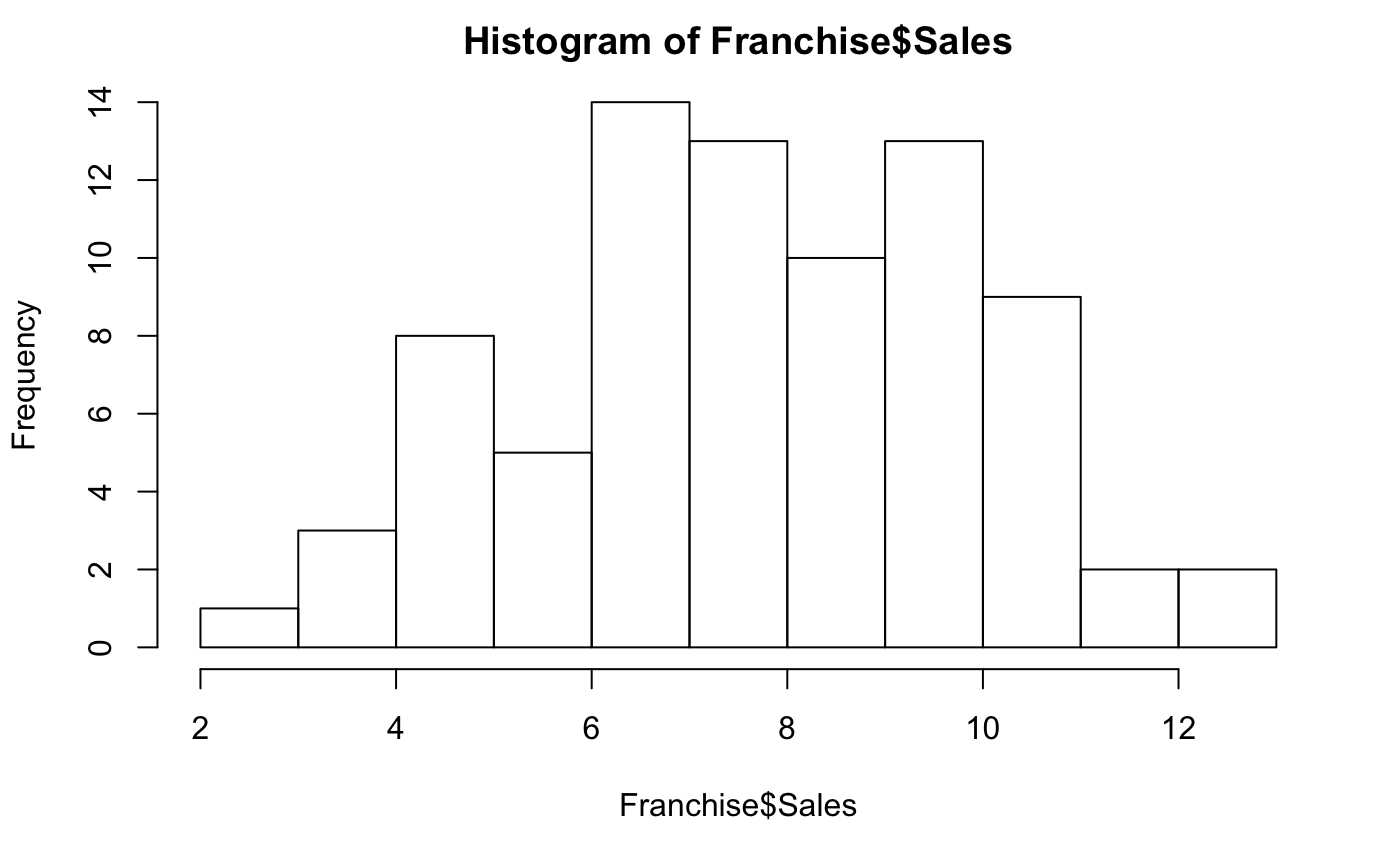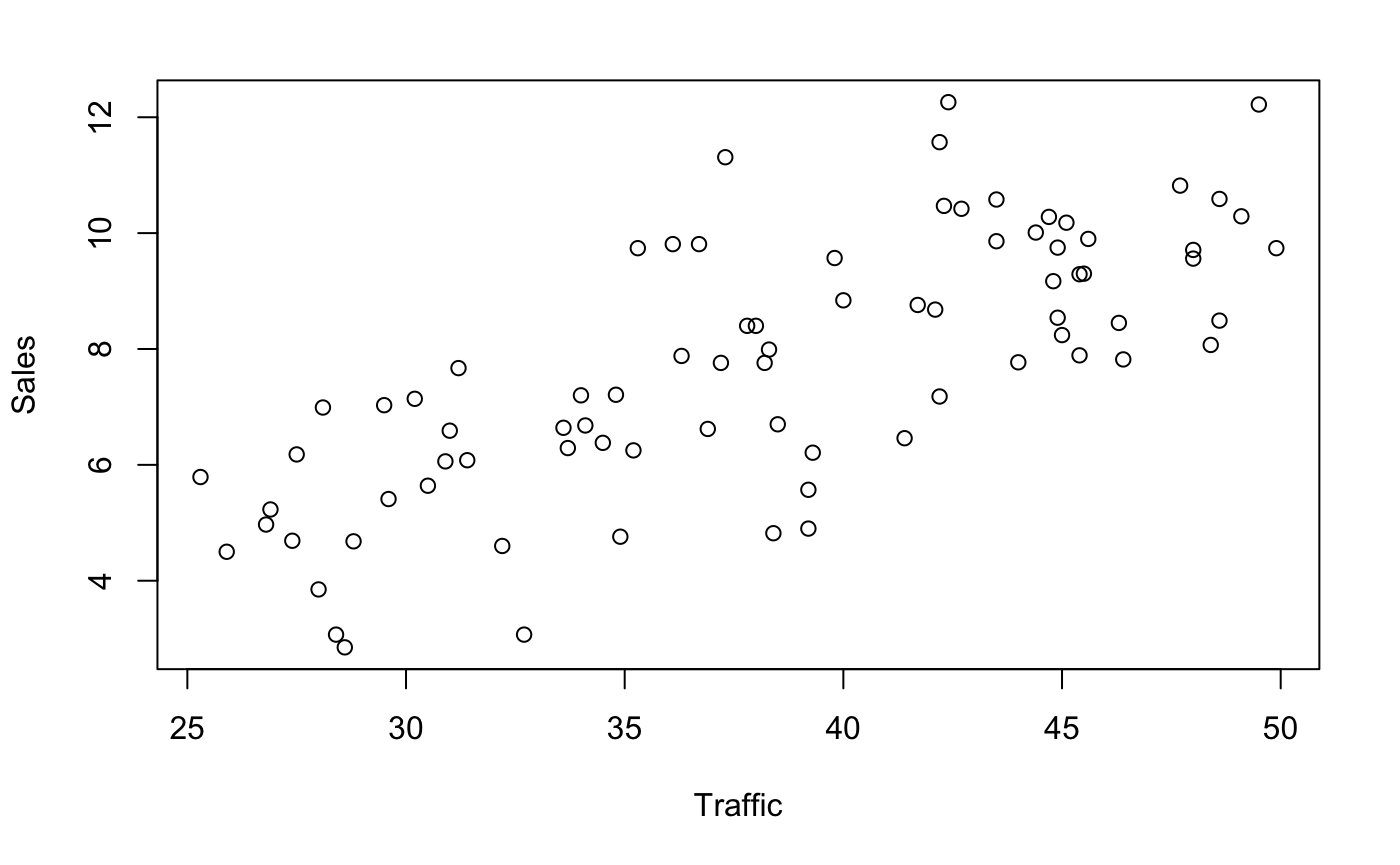This example fits simple regression models to small data sets. The second shows lattice/trellis plots.

library(ggplot2)
library(car)
library(lattice)

To demonstrate the extensibility of R, here’s two functions that allow me to show students the idea of visually testing for association. I would hide the definition of this function from students, but being written in R, the curious students could always find it.

permute <- function(x) { x[sample.int(length(x))] }
visual_test_for_association <- function(x, y, rows=4, cols=5) {
reset()  # compress the margins of plots to fit more together
par(mfrow=c(rows,cols))
ix <- sample.int(rows,1)
iy <- sample.int(cols,1)
for(i in 1:rows) {
for(j in 1:cols) {  # all but one plot shows permuted indices
if( (i==ix)&(j==iy) ) plot(x,y, xlab='x',ylab='y')
else plot(permute(x),y,xlab='x',ylab='y')
}}}

Example 1: Locating a Franchise Outlet

Read the data into a data frame. The data frame has 80 observations of two variables.

Each row of the data frame describes the amount of gasoline sales (in thousands of gallons) and the traffic volume, also in thousands. For example, sales in the first week were 6640 and 7760 in the second week. Traffic volume was 3.3610^{4} in the first week.

Franchise

The View command opens a spreadsheet view of the data. You can only view, not change, the data. Buttons in the header of the view support sorting the columns.

View(Franchise)

Marginal Plots

Histograms are a good starting point, identifying the shape of the distribution and outliers.

hist(Franchise\$Sales, breaks=10)You can then get more clever and add a boxplot to the figure if you’d like, helping to explain both.

hist(Franchise\$Sales, breaks=10)